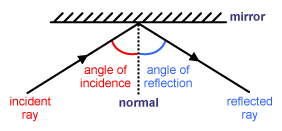A# laws of reflectionReflection of a light ray from a plane mirror.

The two laws of reflection are as follows:

1. The incidenct ray, the reflected ray, and the normal to the reflecting interface at the point of incidence all lie in the same plane.

2. The angle of incidence equals the angle of reflection.

Normal: a line at 90° to the surface of a mirror
Incident ray: A ray of light going to the mirror
Reflected ray: A ray that comes off the mirror
Angle of incidence: The angle between the incident ray and the normal
Angle of reflection: The angle between the reflected ray and the normal